DMT Beauty Transformation: 56 Best Math Trivia Questions And Answers You Should Know

#DMTBeautySpot #beauty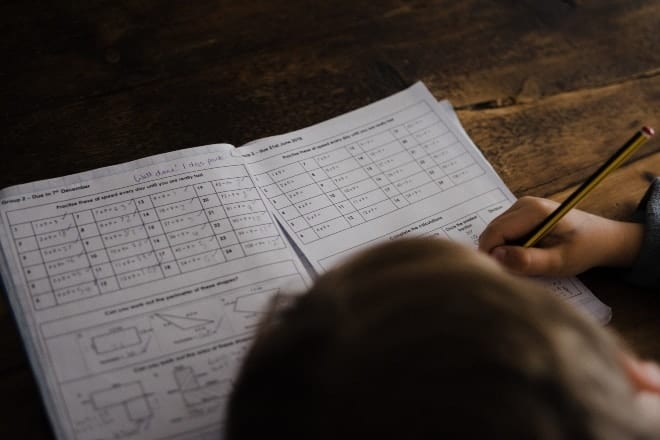via: Unsplash / Annie Spratt

Math trivia is one of those games where you’re able to learn new things in a fun and interesting way. These math trivia questions can be used as a math lesson to help people study or even improve their mathematical skills.

This game of trivia is a great way to get people interested in a topic most find boring or difficult to understand. Even though a lot of these questions are based on mathematical facts rather than problem-solving, they still educate people and get them focused on wanting to learning more about math.

And, if you happen to be someone who enjoys math, then you’ll definitely love learning about these amazing facts that tell you everything there is know about the evolution of mathematics and more.

## 8 Best math trivia questionsvia: Pexels / Roxanne Minnish

Start your game off with only the best math trivia questions that will enlighten you just as much as they educate you. We guarantee you, and anyone else you’re playing with, will find these questions so intriguing that you’ll have a blast throughout the rest of the game.

Here are the 8 best math questions:

#### 1. Lemniscate is the shape of what mathematical symbol?

Infinity

The lemniscate is number 8 on its side. The word comes from the Latin word lemniscatus meaning “ribbon”.

#### 2. What was the number 0 originally called?

Cipher

Cipher comes from the Arabic word sifr meaning “empty”. The first known use of the word zero was in 1598.

#### 3. Who were the first people to ever use negative numbers?

The Chinese

Negative numbers were initially used to figure out the amount of someone’s debt.

#### 4. Most of the mathematical notation in use today was not invented until when?

The 16th century

Before the 16th century, mathematics was written out in words, making mathematical discovery very difficult.

#### 5. What is the study of quantity, structure, space, and change?

Mathematics

The quantity being number theory, the structure being algebra, space being geometry, and change being mathematical analysis.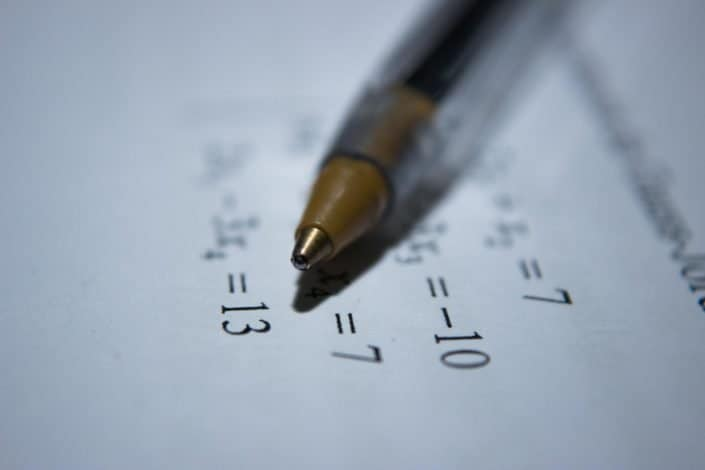via: Unsplash / Antoine Dautry

#### 6. The Fields Medal is often considered a mathematical equivalent to what?

The Nobel Prize

The Fields Medal is made of gold and shows the head of Archimedes along with a quotation attributed to him: (“Rise above oneself and grasp the world”)

#### 7. Where did Archimedes hail from?

Syracuse, Greece

Archimedes was the most famous mathematician and inventor in ancient Greece. He was also known as an astronomer and physicist.

#### 8. How many moves does it take to solve a Rubik’s cube?

no more than 20 moves

It took over 30 years for anyone to figure out that any Rubik’s Cube can be solved in 20 moves and there are 43 quintillion possible configurations of the Cube.

## 10 math trivia questions for kids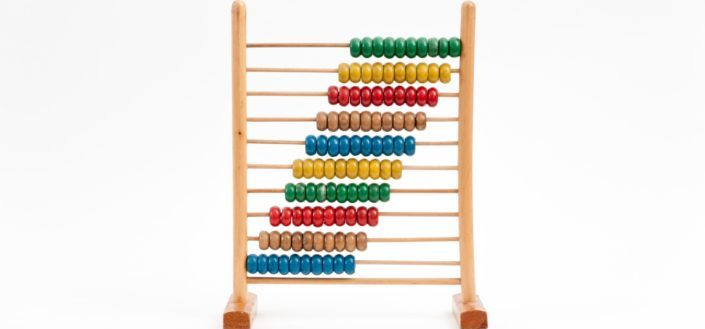via: Unsplash / Crissy Jarvis

Kids don’t always like learning, especially when it comes to a hard subject like math. And some of these trivia questions might be a bit challenging for some age groups of kids. That’s why we’ve put together a list of kids trivia questions that will give them a fun and informative math lesson.

Here are 10 math trivia questions for kids:

#### 9. When is Pi Day celebrated?

43904

It is a day to celebrate the mathematical constant pi ( 3.14) and to eat lots of pie!

#### 10. What is the other name for the International Day of Mathematics?

Pi Day

Interesting fact: the famous astrophysicist Stephen Hawking died on Pi Day, which is coincidentally Albert Einstein’s birth date.via: Pexels / Olya Kobruseva

#### 11. In Israel, instead of “+”, they use what?

inverted “T”

In Israel, the plus sign is missing the bottom stick, making it look like a small inverted “T”.

#### 12. What type of number has no factors other than 1 and itself?

Prime Number

Prime numbers are mostly odd except for the number 2, which is used as factors for almost every composite number.

#### 13. What number is represented in Roman Numerals by the letter ‘D’?

500

Letters were commonly used to represent numbers in ancient Rome.

I = 1; V = 5; X = 10; L = 50; C = 100; D = 500; M = 1000

#### 14. What do you call an angle more than 90 degrees and less than 180 degrees?

Obtuse

Some objects that depict obtuse angles are a book (when opened to certain angles), a pair of scissors, and a door opened wide.

#### 15. What’s a polygon with four unequal sides called?

Some examples of a quadrilateral are the shapes; Square, Rectangle, Rhombus, Trapezoid, and Parallelogram.via: Pexels / Athena

#### 16. What’s the top number of a fraction called?

the numerator

The numerator tells how many equal parts of the whole, or collection, are taken from the denominator (bottom part of a fraction).

#### 17. What number is an improper fraction always greater than?

one

With improper fractions, the numerator is always larger than the denominator.

#### 18. What is a polygon with eight sides?

Octagon

Oct is a representation of the number 8, as in octopus and October. October was originally the 8th month of the year in the old calendar of Romulus.

## 8 math trivia questions for teensvia: Pexels / Jeswin Thomas

Our round of kids trivia might not be challenging enough for those in higher levels. So, to even things out, use these math trivia questions for teens to make sure you’re able to include all age groups in this game of math trivia.

Here are 8 math trivia questions for teens:

#### 19. Where were the Arabic numerals invented?

India

What’s interesting is that the English numbers used today are actually Arabic numerals from centuries ago.

#### 20. What is the math term described as the likelihood that something will happen?

Probability

Probability is a mathematical term that we use in our everyday lives to determine the chances of something happening.via: Pexels / Christina Morillo

#### 21. What common math term means “for every one hundred” in Latin?

percent

Fractions, ratios and decimals are also parts taken for every one hundred.

#### 22. What is the name given to a triangle with all sides of a different length?

Scalene

A scalene triangle also has different measurements of each angle.

#### 23. What may be mixed, complex or vulgar?

Fractions

In other terms, fractions can be mixed numbers/fractions, improper fractions, and simple fractions.

#### 24. What was developed as a tool used to investigate change in natural sciences?

Calculus

Calculus is used in many different areas such as physics, astronomy, biology, engineering, economics, medicine and sociology.

#### 25. The most notable achievement of Islamic mathematics was the development of what?

Algebra

Other achievements included the use of decimals, mathematical induction, and trigonometry.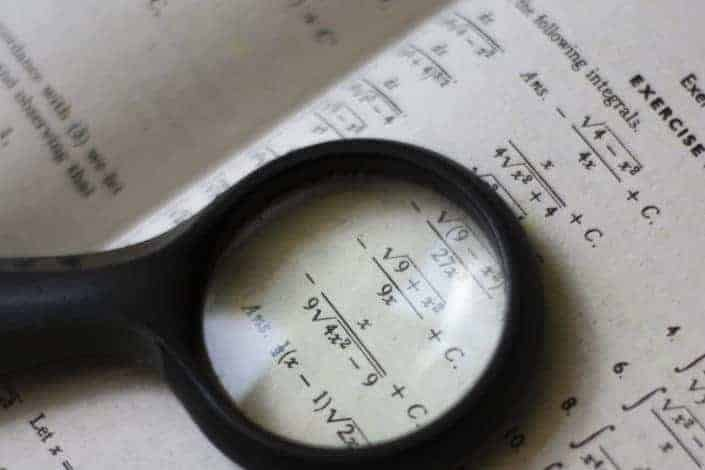via: Pexels / Ian Panelo

#### 26. What mathematician noticed that the morning and evening star were one and the same?

Pythagoras

This morning and evening star was discovered to be the planet Venus, which is visible to earth at certain times of the day.

## 9 fun math trivia questions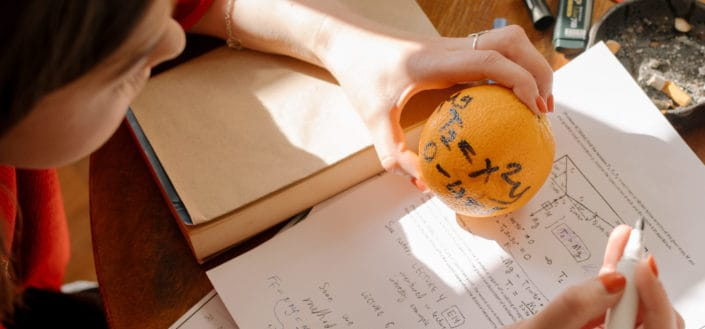via: Pexels / cottonbro

Math can actually be very exciting when you understand what you’re learning, and with these fun trivia questions you’ll learn about the most amusing math facts that you’ve ever come across.

Here are 9 fun math trivia questions:

#### 27. What unit of time is 1/100th of a second?

jiffy

Now you know why people use the phrase “in a jiffy” to refer to doing something very quickly.

#### 28. Every odd number has what letter in it?

e:

The letter E is a pretty common vowel, so it’s not odd that it’s present in every odd number. What is odd is that it’s not in every even number as well.

#### 29. Convert -40 °C to Fahrenheit.

-40 °F

This is the only time you’ll see Celsius and Fahrenheit converted to the same degree temperatures.via: Unsplash / Jarosław Kwoczała

#### 30. How many sides does an “Enneadecagon” have?

19

This is a 19 Sided polygon that’s pretty similar in shape to a circle.

#### 31. What is the first number to contain the letter ‘a’?

one thousand

Many other English speaking countries would argue that the first number with the letter ‘a’ is 101, due to the fact that it’s said “one hundred And one”.

#### 32. What is the only number to have its letters in alphabetical order?

Forty

While the number ‘one’ is the only number in which the letters are in reverse alphabetical order.

#### 33. What number comes first if all numbers are arranged alphabetically?

8

The letter ‘E’ is a pretty popular letter among the numbers. It’s present in most numbers and makes the number 8 come first alphabetically. Also, 0 would come last when arranged in alphabetical order.

#### 34. What is the only number that is twice the sum of its digits?

18

8 plus 1 equals 9. 9 plus 9 equals 18.

#### 35. How many digits of pi are there?

Infinity

The record for finding consecutive numbers, from 3.14 onward to the final digit, is held by Fabrice Bellard, who announced that he had calculated pi to 2.7 trillion digits.

## 8 easy math trivia questionsvia: Unsplash / Chris Liverani

Everyone knows math is a tough subject. Lots of people have a hard time understanding many math concepts. For those people, we’ve gathered some of the most easy trivia questions to help make learning about math all the more easier.

Here are 8 easy math trivia questions:

#### 36. In Chinese and Japanese cultures, the number 4 is associated with what?

death

Number 4 is considered unlucky because it sounds close to the Chinese word meaning death. In China, you can find several buildings without a 13th and 4th floor because of how unlucky these numbers are.via: Pexels / Anand Raghu

#### 37. What is the symbol of division called?

obelus

A division sign can also be represented by a slash(/) or a horizontal line, much like you would see on a fraction.

#### 38. What is the name for the longest side of a right triangle?

Hypotenuse

The hypotenuse of a right triangle is always the side opposite the right angle.

#### 39. What are the first four digits of Pi?

3.141

There are many people who go on to memorize as many digits of Pi as they’re able to remember. That’s pretty tough, considering the digits of Pi are infinite.

#### 40. How many degrees in a full circle?

360

The understanding of why a circle is based on parts of 60 is due to the Babylonians idea of a year being close to 360 days.via: Unsplash / Austin Neill

#### 41. What is the name for a 3 sided polygon?

Triangle

There are a number of different types of triangles, such as equilateral triangles, right triangles, scalene triangles, obtuse triangles, acute triangles, and isosceles triangles.

#### 42. What is the only number that has the same number of letters?

FOUR

Even though this number is considered unlucky in some parts of the world, this fact right here makes it pretty difficult to think so.

#### 43. What number is the only even prime number?

2

This is likely due to the small amount of the number 2. What’s interesting is most composite numbers use the number 2 as one of its divisors.

## 7 random math trivia questionsvia: Unsplash / Joshua Hoehne

For this round, we’ve given you random trivia questions and answers based on math facts that have no specific pattern, whatsoever. So, get ready to answer just about any question pertaining to math!

Here are 7 random math trivia questions:

#### 44. What had a simple calculation error that went unnoticed for 300 years?

Principia Mathematica

In 1987, a 23 year old physics student discovered that Sir Issac Newton plugged the wrong value into a calculation.

#### 45. In a group of 23 people, what is it called when there’s a 50% chance that at least two will have the same birthday?

By the pigeonhole principle, the probability reaches 100% when the number of people reaches 367.via: Unsplash / Andreas Weiland

#### 46. What number does not have its own Roman numeral?

zero

This is because the Roman’s did not use, or have a need for the number 0.

#### 47. In a recent online poll of 3,000 people by Alex Bellos, what is the most people’s favorite number?

7

The number 7 is seen as the center of humanity and its connection to the creator, not to mention all the luck that’s commonly associated with this number.

#### 48. What is the old Norse term from which the word “hundred” came from?

Hundrath

The old Norse term hundrath actually means 120.

#### 49. What is the meaning of ‘crore’?

10 million

It’s an Indian term especially referred to rupees, units of measurement, or people.

#### 50. What is the smallest number that can be exactly divided by all the numbers 1 to 10?

2520

Now that you know this, try to calculate the smallest number that’s divisible by the numbers 1-20!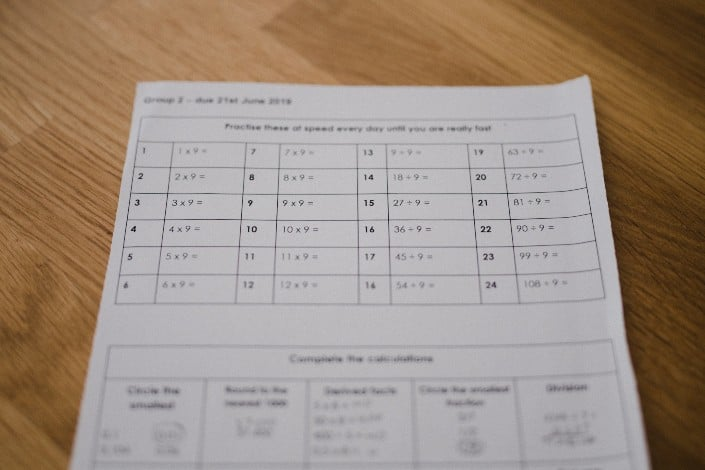via: Unsplash / Anni Spratt

## 6 hard math trivia questionsvia: Pexels / RF._.studio

For those people who claim to know so much about mathematics and love solving any type of math problems, give them a challenge they can’t resist with these hard trivia questions. Just make sure to let them know that these questions are pretty tough to answer!

Here are 6 hard math trivia questions:

#### 51. Markings on animal bones indicate that humans have been doing math since when?

30,000 BC

This animal bone is called the Ishango Bone and has Tally marks carved along it.

#### 52. Which ancient mathematician used the value of zero for the first time?

Brahmagupta

Brahmagupta was also the first to show that subtracting a number from itself results in zero.

#### 53. What did John Napier invent in 1614?

Logarithms 50

He also invented the so-called “Napier’s bones” and made common the use of the decimal point in arithmetic and mathematics.

#### 54. How many equal sides does an icosahedron have?

Twenty

An icosahedron is a complex shape containing 30 edges and 20 equilateral triangle faces with five meeting at each of its twelve vertices.

#### 55. Who invented the equals sign?

Robert Recorde

When writing his book, Robert Recorde wrote the phrase ‘is equal to’ more than 200 times. To make things sound less repetitive, he changed ‘is equal to’ to the equals sign we all use today.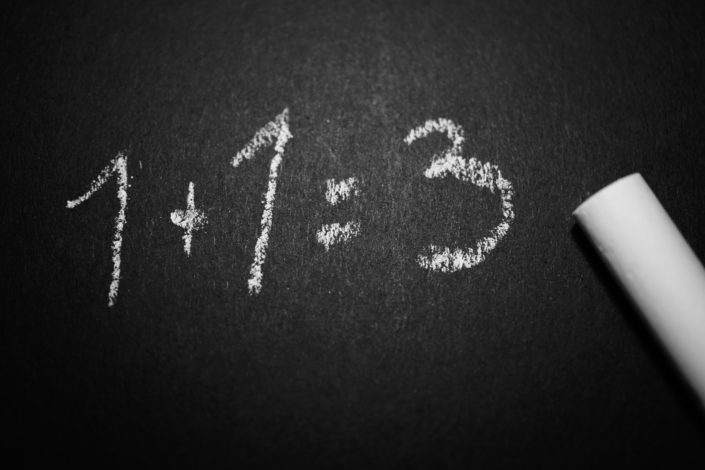via: Pexels / George Becker

#### 56. What algebraic system is one of the legacies of Rene Descartes?

Cartesian geometry

This type of geometry is used with Coordinate systems, which can be displayed on the X and Y axis.

Here is a downloadable list of math trivia questions (right-click the image and select Save Image As:..)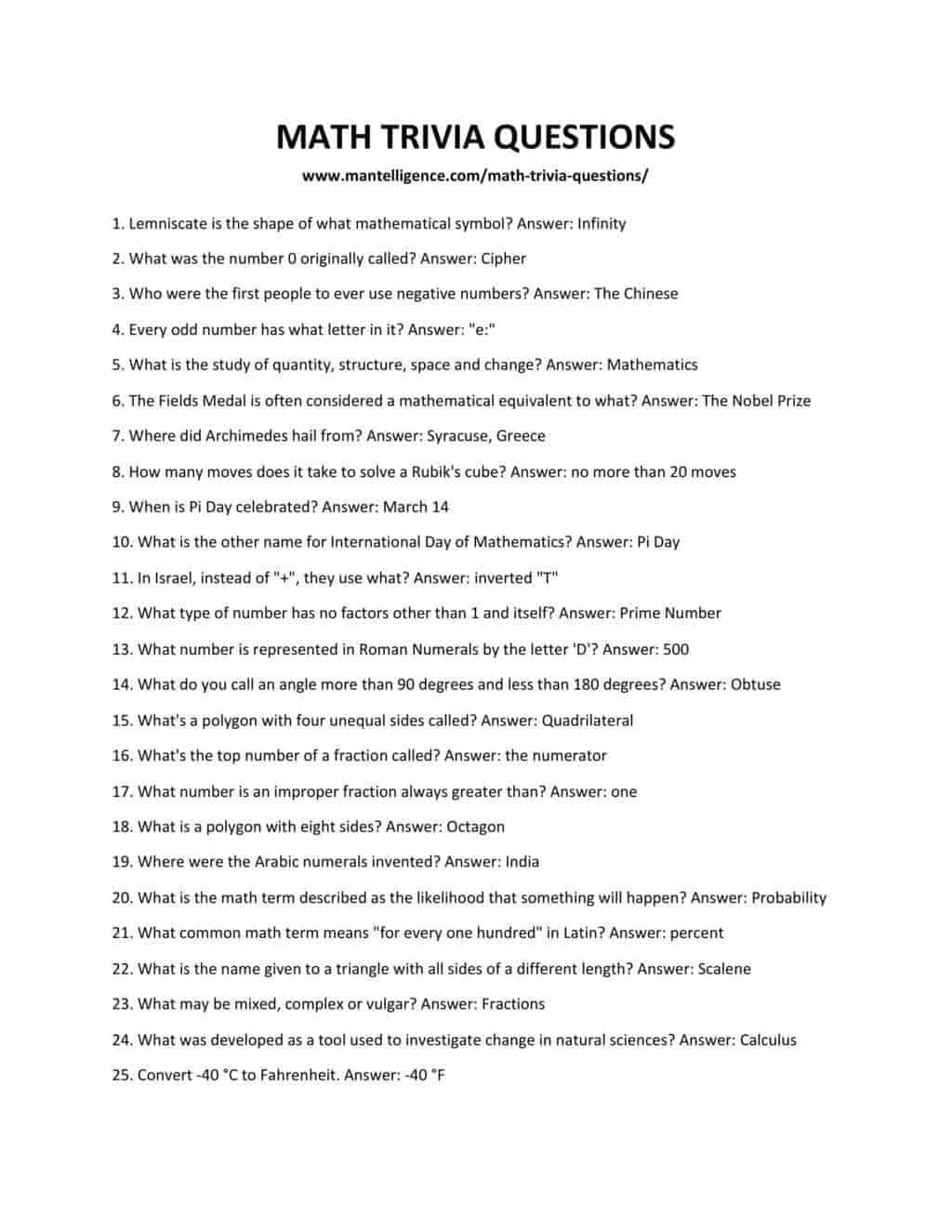via: Mantelligence

## More Awesome Trivia Questions

Love learning new facts with these academic based trivia games? Then try out some more trivia subjects that will allow you to have fun while also learning a thing or two.

1. Science trivia, much like math trivia, is a great way to brush up on school subjects in a fun and easy way.
2. If you’re a sports fan then you’ll love these sports trivia questions on all your favorite sports.
3. Another great game to get your brain pumping is geography trivia. Learn about all the great geography facts this world has to offer.

## How To Pick The best math trivia questions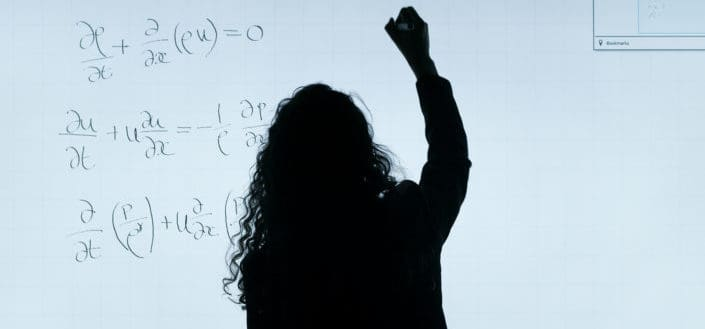via: Pexels / ThisIsEngineering

Choosing the best math trivia questions for your game of trivia can be a bit overwhelming. That’s why there’s a few things you should consider before deciding which questions will give you an exciting game of math trivia.

Here is how to pick the best math trivia questions:

1. Keep the players’ ages in mindvia: Pexels / Max Fischer

When it comes to math trivia, you need to be aware of the age groups of the people who will be playing the game. If you’re planning on playing with young kids, choose questions that you think they should know. Figure out what they’re learning about in their math classes and choose questions that fall in those levels.

On the other hand, if you’re playing with college students, don’t be afraid to mix the questions up with a range of easy and hard choices.

2. Choose fun and interesting questionsvia: Unsplash / Cleo Vermij

Math isn’t a fun subject for everyone, so if you plan on playing with people who aren’t that into math, keep the game going by adding lots of fun and interesting questions. There are plenty of interesting math facts on this list. Just pick the ones that you think will be fun for others to learn about.

3. Select a few questions that are prize-worthyvia: Unsplash / Ariel

If you’re really trying to get people excited to play a game of math trivia, give out prizes for questions answered correctly. These can be questions that you feel are a bit challenging or it can be completely random. Either way, prizes are a great way to get players eagerly participating.

## In Conclusion

Whether you’re planning to play this game of math trivia for leisure or for a fun math lesson, remember that the players will benefit either way. Trivia questions are the perfect way to get the brain working and a fun way to learn new and amazing facts.

So, go ahead and gather everyone you know for this fun and fact filled game of math trivia. We guarantee you’ll have a blast!

The post 56 Best Math Trivia Questions And Answers You Should Know appeared first on Mantelligence.

DMTBeautySpot

via https://www.DMTBeautySpot.com

Jasper, Khareem Sudlow